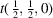International
Tables for
Crystallography
Volume A
Space-group symmetry
Edited by M. I. Aroyo

International Tables for Crystallography (2016). Vol. A, ch. 2.1, pp. 161-162

## Section 2.1.3.10. Generators

Th. Hahna and A. Looijenga-Vosb

#### 2.1.3.10. Generators

| top | pdf |

The line Generators selected states the symmetry operations and their sequence, selected to generate all symmetry-equivalent points of the General position from a point with coordinates x, y, z. Generating translations are listed as t(1, 0, 0), t(0, 1, 0), t(0, 0, 1); likewise for additional centring translations. The other symmetry operations are given as numbers (p) that refer to the corresponding coordinate triplets of the general position and the corresponding entries under Symmetry operations, as explained in Section 2.1.3.9[for centred space groups the first block For (0, 0, 0)+ set' must be used].

For all space groups, the identity operation given by (1) is selected as the first generator. It is followed by the generators t(1, 0, 0), t(0, 1, 0), t(0, 0, 1) of the integral lattice translations and, if necessary, by those of the centring translations, e.g.for a C-centred lattice. In this way, point x, y, z and all its translationally equivalent points are generated. (The remark and its translationally equivalent points' will hereafter be omitted.) The sequence chosen for the generators following the translations depends on the crystal class of the space group and is set out in Table 1.4.3.1.

#### Example:(14, unique axis b, cell choice 1)

After the generation of (1) x, y, z, the operation (2) which stands for a twofold screw rotation around the axis 0, y,generates point (2) of the general position with coordinate triplet. Finally, the inversion (3) generates point (3)from point (1), and point (4′)from point (2). Instead of (4′), however, the coordinate triplet (4)is listed, because the coordinates are reduced modulo 1.

The example shows that for the space grouptwo operations, apart from the identity and the generating translations, are sufficient to generate all symmetry-equivalent points. Alternatively, the inversion (3) plus the glide reflection (4), or the glide reflection (4) plus the twofold screw rotation (2), might have been chosen as generators. The process of generation and the selection of the generators for the space-group tables, as well as the resulting sequence of the symmetry operations, are discussed in Section 1.4.3.

The generating operations for different descriptions of the same space group (settings, cell choices, origin choices) are chosen in such a way that the transformation relating the two coordinate systems also transforms the generators of one description into those of the other (cf. Section 1.5.3).